Logarithms

 Site: TBAISD Moodle Course: Michigan Algebra II Preview 2012 Book: Logarithms Printed by: Guest user Date: Friday, July 30, 2021, 07:34 PM

Method 1

There are many different types of logarithmic equations. The first type uses properties to convert more than one log into a single log expression and then applies inverses to solve.

Example 1 Solve log3 4 + log3 x = 1

Step 1. Use properties to convert to a single log.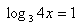Step 2. Translate the log equation to its equivalent exponential form.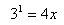Step 3. Solve for x.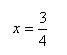Example 2

Solve the equation log 43 - log 412 = x
Step 1. Use properties to convert to a single log.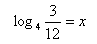Step 2. Translate the log equation to its equivalent exponential form.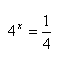Step 3. If needed, change the two expressions to the same base and solve for x.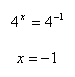Method 2

Another type of log equation is one where there is a log on both sides of the equal sign. To solve this type of equation, use the inverse relationship between logs and exponents.

Example 1 Solve the equation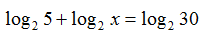Step 1. Use Product of a Power Property to convert to a single log.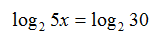Step 2. Use the inverse operation of exponents on both sides of the equals sign. Since the log has a base of 2, use an exponential base of 2.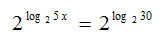Step 3. Since logs and exponents are inverses, the exponent base and the log base cancel.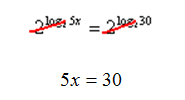Step 4 . Solve for x.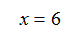Example 2

Solve the equation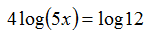Step 1. Use Power of a Power Property to convert to a single log.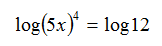Step 2. Use the inverse operation of exponents on both sides of the equals sign. Since the common log has a base of 10, use an exponential base of 10.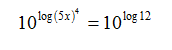Step 3. Since logs and exponents are inverses, the exponent base and the log base cancel.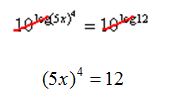Step 4 . Solve for x.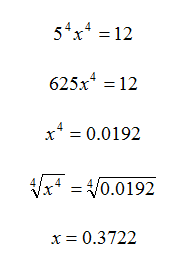Interactive Activitiy

Logarithm Self-Check Quiz

Video Lesson

To learn how to solve log equations, select the following link:

Solving Logarithmic Equations

Guided Practice

To solidify your understanding of evaluating exponential and logarithmic equations, visit the following link to Holt, Rinehart, and Winston Homework Help Online. It provides examples, video tutorials, and interactive practice with answers available. The Practice and Problem Solving section has two parts. The first part offers practice with a complete video explanation for the type of problem with just a click of the video icon. The second part offers practice with the solution for each problem only a click of the light bulb away.

Practice

Solving Logarithms Worksheet